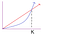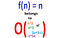# Big O Notation In Plain English

This article aims to simplify Big-O Notation and what it means. Big O Notation can sometimes seem complicated, many definitions especially in academics can be hard for students to understand. I hope that in this article I can simplify the definition for you.

# Formal Definition of Big O

First let’s look at the formal definition. Big O notation is a mathematical notation that describes the limiting behavior of a function when the argument tends towards a particular value or infinity, and is usually written as ‘O’. It is an Asymptotic Notation for the worst case, which is also the ceiling of growth for a given function. It provides us with what is called an asymptotic upper bound for the growth rate of runtime of an algorithm or program.

The mathematical definition is below:
`f(n) is O(g(n)) if and only if f(n) <= C * g(n) for all n >= k, where C and k are positive constant values`At point K, the blue line g(n) grows faster than the red line f(n)

# Simplified Definition of Big O

That is a handful of information for describing Big O notation. Simply put Big O is a set of functions that are all limiting some other function(s), meaning the function(s) will never grow faster than the other functions that are in the set at a specific point.

Here is an example, n is O(n²). This is a true statement, but why ?
This is because the function f(n) = n, will never exceed or grow faster than the function g(n) = n², no matter what positive value you input for the variable ’n’, n² will always be greater. The specific point where the function n² is greater than or equal to ’n’ is when ’n’ is equal to 1.

Mathematically :
`n is O(n^2) if and only if n <= 1* n^2 for all n >= 1, where C=1 and k=1 which are positive constant values`Example of a few functions that f(n) belongs to in Big O Notation.

# Big O Notation Examples

Suppose `f(n)` is your algorithm runtime, and `g(n)` is an arbitrary time complexity you are trying to relate to your algorithm. `f(n)` is O(g(n)), if for some positive constants c and k, `f(n)` <= `c * g(n)` whenever n ≥ k

# Prove Big-O (O)

Lets take a look at an example:
You can look at the following video instead of reading this or use it as extra material, since I will prove the following within this article as well. In the video below, we want to show that f(n) is Big O of g(n).

f(n) = n² + 2n + 1
g(n) = n²

So we want to show/prove that n² + 2n + 1 is O(n²)

Lets try and solve this. First we have f(n) = n² + 2n + 1 and g(n) = n², we therefore must show f(n) <= c * g(n) whenever n ≥ k, that is
n² + 2n + 1 ≤ c * n² whenever n > k.

Since k must be a positive integer (greater than or equal to 1) lets set it equal to 1 to get the following: n² + 2n + 1 ≤ c * n² whenever n ≥ 1.

Next we can do one of two things, either solve for our constant c > 0 or find a constant c >0 . Like the video above, we will solve for c, by rewriting our original equation to get: (n² + 2n + 1 ) / n² ≤ c whenever n ≥ 1.

NOTE: (n² + 2n + 1 ) / n² ≤ (n² + 2n² + n²) / n² whenever n > 1, therefore:
(n² + 2n + 1 ) / n² ≤ (n² + 2n² + n²) / n² ≤ c whenever n ≥ 1.

So (n² + 2n² + n²) / n² ≤ c whenever n ≥ 1. Lets solve this equation for our constant c.

=(n² + 2n² + n²) / n² ≤ c whenever n ≥ 1
=(1 + 2 + 1) / 1 ≤ c whenever n ≥ 1
= 4 ≤ c whenever n ≥ 1
= c = 4

Okay so now we have our constant c=4, lets plug it back into our original equation to get: n² + 2n + 1 ≤ 4* n² whenever n ≥1 and you will find that for any value of n greater than 1 this equation is always true.

So f(n) is O(g(n) ), that is n² + 2n + 1 is O(n²).

# Big O Example Using Code

Now that we understand what Big O is and how to prove it, use the video below called Big O Example to figure out the running time of the following code snippet. The answer is O(n⁵), but the video will explain how.

# Video of Big O Example Using Code

Thanks for reading this article I hope its helpful to you all ! Keep up the learning, and if you would like more algorithm analysis videos please visit and subscribe to my YouTube channels (randerson112358 & compsci112358 )

# Check Out the following for more content / videos on Algorithm Analysis and Programming:

compsci112358:

Video Tutorials on Recurrence Relation:

Video Tutorial on Algorithm Analysis:
https://www.udemy.com/algorithm-analysis/

Written by